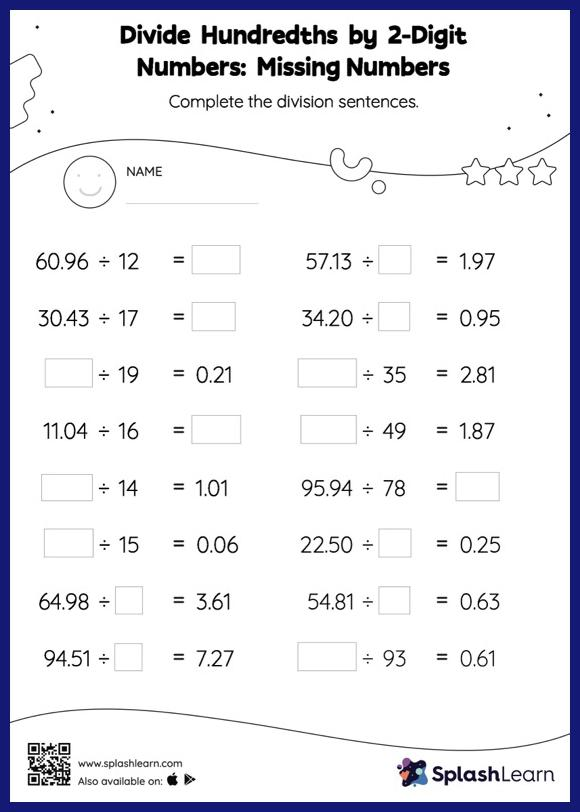# Divide Hundredths by 2-Digit Numbers without Remainder: Missing Numbers Worksheet

Home > Divide Hundredths by 2-Digit Numbers without Remainder: Missing NumbersWhen dividing decimals by 1-digit numbers, students first divide the same way they would divide with whole numbers. Then they add a decimal point at the correct place in the quotient depending on the number of digits in the dividend. Divide hundredths by 2-digit numbers without remainder worksheet helps them improve accuracy with this concept as they find the missing number in decimal division. This worksheet is about practicing with the horizontal format in which numbers are written side by side. To develop flexibility with numbers and operations, students need to have enough practice in this format and not just rely on the vertical/column method.